# Keras 图像增强

## Keras图像增强API

Keras的图像增强(image augmentation) API简单而强大。

Keras提供ImageDataGenerator类别，用于定义图像数据准备和增强的配置。这包括以下功能：

• 图像随机旋转(rotate)
• 图像随机偏移(shift)
• 图像随机推移错切(shear)
• 图像随机翻转(flip)
• Sample-wise 图像像素标准化
• Feature-wise 图像像素标准化
• ZCA 白化转换
• 图像张量维度的重排序
• 储存增强图像数据
• 增强图像生成器可以透过以下的方法创建：
datagen = ImageDataGenerator()


Keras并不是在记忆体中对整个图像数据集执行图像转换操作，而是设计为通过深度学习模型训练过程进行迭代，从而为您动态地创建增强的图像数据。这会减少您的记忆体开销，但在模型训练期间会增加一些额外的时间成本。

datagen.fit(train)


X_batch, y_batch = datagen.flow(train, train, batch_size=32)


model.fit_generator(datagen.flow(X_train, Y_train, batch_size=batch_size),
steps_per_epoch=len(X_train)/batch_size,
epochs=nb_epoch)


## 图像增强(Image Augmentation)相关参数的比较

import  keras
# Plot images
from  keras.datasets  import  mnist
import  matplotlib.pyplot  as  plt

( X_train ,  y_train ),  ( X_test ,  y_test )  =  mnist . load_data ()

plt . figure ( figsize = ( 8 , 8 ))  #设定每个图像显示的大小

#产生一个3x2网格的组合图像
for  i  in  range ( 0 ,  6 ):
plt . subplot ( 330 + 1 + i )  # (331) ->第一个子图像, (332) ->第二个子图像
plt . title ( y_train [ i ])
plt . axis ( 'off' )      #不显示坐标
plt . imshow ( X_train [ i ],  cmap = plt . get_cmap ( 'gray' )) # 以灰阶的图像显示

#展现出图像
plt . show ()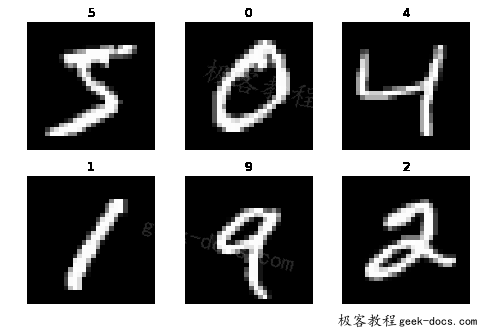## 随机旋转(Random Rotations)

#随机旋转(Random Rotations)
from  keras.datasets  import  mnist
from  keras.preprocessing.image  import  ImageDataGenerator
import  matplotlib.pyplot  as  plt

#载入数据
( X_train ,  y_train ),  ( X_test ,  y_test )  =  mnist . load_data ()

#将图像数据集的维度进行改变
#改变前: [样本数,图像宽,图像高] ->改变后: [样本数,图像宽,图像高,图像频道数]
X_train  =  X_train . reshape ( X_train . shape [ 0 ],  28 ,  28 ,  1 )
X_test   =  X_test . reshape ( X_test . shape [ 0 ],  28 ,  28 ,  1 )

#将像素值由"整数(0~255)"换成"浮点数(0.0~255.0)"
X_train  =  X_train . astype ( 'float32' )
X_test  =  X_test . astype ( 'float32' )

#定义"图像数据增强产生器(ImageDataGenerator)"的参数
datagen  =  ImageDataGenerator ( rotation_range = 90 )

#透过训练数据集来训练(fit)图像数据增强产生器(ImageDataGenerator)的实例
datagen . fit ( X_train )

#设定要"图像数据增强产生器(ImageDataGenerator)"产生的图像批次值(batch size)
# "图像数据增强产生器(ImageDataGenerator)"会根据设定回传指定批次量的新生成图像数据
for  X_batch ,  y_batch  in  datagen . flow ( X_train ,  y_train ,  batch_size = 9 ):
plt . figure ( figsize = ( 8 , 8 ))  #设定每个图像显示的大小
#产生一个3x3网格的组合图像
for  i  in  range ( 0 ,  6 ):
plt . subplot ( 331+ i )
plt . title ( y_batch [ i ])  #秀出图像的真实值
plt . axis ( 'off' )      #不显示坐标
plt . imshow ( X_batch [ i ] . reshape ( 28 , 28 ),  cmap = plt . get_cmap ( 'gray' ))

plt . show ()
break  #跳出回圈## 随机偏移(Random Shifts)

#随机偏移(Random Shifts)
from  keras.datasets  import  mnist
from  keras.preprocessing.image  import  ImageDataGenerator
import  matplotlib.pyplot  as  plt

#载入数据
( X_train ,  y_train ),  ( X_test ,  y_test )  =  mnist . load_data ()

#将图像数据集的维度进行改变
#改变前: [样本数,图像宽,图像高] ->改变后: [样本数,图像宽,图像高,图像频道数]
X_train  =  X_train . reshape ( X_train . shape [ 0 ],  28 ,  28 ,  1 )
X_test   =  X_test . reshape ( X_test . shape [ 0 ],  28 ,  28 ,  1 )

#将像素值由"整数(0~255)"换成"浮点数(0.0~255.0)"
X_train  =  X_train . astype ( 'float32' )
X_test  =  X_test . astype ( 'float32' )

#定义"图像数据增强产生器(ImageDataGenerator)"的参数
shift  =  0.2
datagen  =  ImageDataGenerator ( width_shift_range = shift ,  height_shift_range = shift )

#透过训练数据集来训练(fit)图像数据增强产生器(ImageDataGenerator)的实例
datagen . fit ( X_train )

#设定要"图像数据增强产生器(ImageDataGenerator)"产生的图像批次值(batch size)
# "图像数据增强产生器(ImageDataGenerator)"会根据设定回传指定批次量的新生成图像数据
for  X_batch ,  y_batch  in  datagen . flow ( X_train ,  y_train ,  batch_size = 9 ):
plt . figure ( figsize = ( 8 , 8 ))  #设定每个图像显示的大小
#产生一个3x3网格的组合图像
for  i  in  range ( 0 ,  6 ):
plt . subplot ( 331+ i )
plt . title ( y_batch [ i ])  #秀出图像的真实值
plt . axis ( 'off' )      #不显示坐标
plt . imshow ( X_batch [ i ] . reshape ( 28 , 28 ),  cmap = plt . get_cmap ( 'gray' ))

plt . show ()
break  #跳出回圈## 随机推移错切(Random Shear)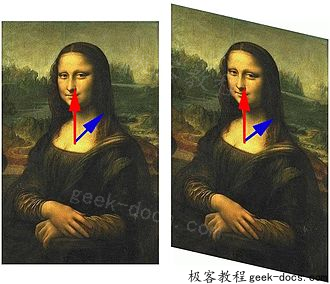Keras的”图像数据增强产生器(ImageDataGenerator)”支持使用shear_range参数设定来对训练数据进行图像错切变换来产生新图像数据。

#随机推移错切(Random Shear)
from  keras.datasets  import  mnist
from  keras.preprocessing.image  import  ImageDataGenerator
import  matplotlib.pyplot  as  plt

#载入数据
( X_train ,  y_train ),  ( X_test ,  y_test )  =  mnist . load_data ()

#将图像数据集的维度进行改变
#改变前: [样本数,图像宽,图像高] ->改变后: [样本数,图像宽,图像高,图像频道数]
X_train  =  X_train . reshape ( X_train . shape [ 0 ],  28 ,  28 ,  1 )
X_test   =  X_test . reshape ( X_test . shape [ 0 ],  28 ,  28 ,  1 )

#将像素值由"整数(0~255)"换成"浮点数(0.0~255.0)"
X_train  =  X_train . astype ( 'float32' )
X_test  =  X_test . astype ( 'float32' )

#定义"图像数据增强产生器(ImageDataGenerator)"的参数
shear_range = 1.25  #推移错切的强度
datagen  =  ImageDataGenerator ( shear_range = shear_range )

#透过训练数据集来训练(fit)图像数据增强产生器(ImageDataGenerator)的实例
datagen . fit ( X_train )

#设定要"图像数据增强产生器(ImageDataGenerator)"产生的图像批次值(batch size)
# "图像数据增强产生器(ImageDataGenerator)"会根据设定回传指定批次量的新生成图像数据
for  X_batch ,  y_batch  in  datagen . flow ( X_train ,  y_train ,  batch_size = 9 ):
plt . figure ( figsize = ( 8 , 8 ))  #设定每个图像显示的大小
#产生一个3x3网格的组合图像
for  i  in  range ( 0 ,  6 ):
plt . subplot ( 331+ i )
plt . title ( y_batch [ i ])  #秀出图像的真实值
plt . axis ( 'off' )      #不显示坐标
plt . imshow ( X_batch [ i ] . reshape ( 28 , 28 ),  cmap = plt . get_cmap ( 'gray' ))

plt . show ()
break  #跳出回圈## 随机镜像翻转(Random Flips)

Keras的”图像数据增强产生器(ImageDataGenerator)”支持使用vertical_flip和horizo​​ntal_flip两个参数设定来对训练数据进行垂直和水平轴随机翻转来产生新图像数据。

#随机镜像翻转(Random Flips)
from  keras.datasets  import  mnist
from  keras.preprocessing.image  import  ImageDataGenerator
import  matplotlib.pyplot  as  plt

#载入数据
( X_train ,  y_train ),  ( X_test ,  y_test )  =  mnist . load_data ()

#将图像数据集的维度进行改变
#改变前: [样本数,图像宽,图像高] ->改变后: [样本数,图像宽,图像高,图像频道数]
X_train  =  X_train . reshape ( X_train . shape [ 0 ],  28 ,  28 ,  1 )
X_test   =  X_test . reshape ( X_test . shape [ 0 ],  28 ,  28 ,  1 )

#将像素值由"整数(0~255)"换成"浮点数(0.0~255.0)"
X_train  =  X_train . astype ( 'float32' )
X_test  =  X_test . astype ( 'float32' )

#定义"图像数据增强产生器(ImageDataGenerator)"的参数
datagen  =  ImageDataGenerator ( horizo​​ntal_flip = True ,  vertical_flip = True )

#透过训练数据集来训练(fit)图像数据增强产生器(ImageDataGenerator)的实例
datagen . fit ( X_train )

#设定要"图像数据增强产生器(ImageDataGenerator)"产生的图像批次值(batch size)
# "图像数据增强产生器(ImageDataGenerator)"会根据设定回传指定批次量的新生成图像数据
for  X_batch ,  y_batch  in  datagen . flow ( X_train ,  y_train ,  batch_size = 9 ):
plt . figure ( figsize = ( 8 , 8 ))  #设定每个图像显示的大小
#产生一个3x3网格的组合图像
for  i  in  range ( 0 ,  6 ):
plt . subplot ( 331+ i )
plt . title ( y_batch [ i ])  #秀出图像的真实值
plt . axis ( 'off' )      #不显示坐标
plt . imshow ( X_batch [ i ] . reshape ( 28 , 28 ),  cmap = plt . get_cmap ( 'gray' ))

plt . show ()
break  #跳出回圈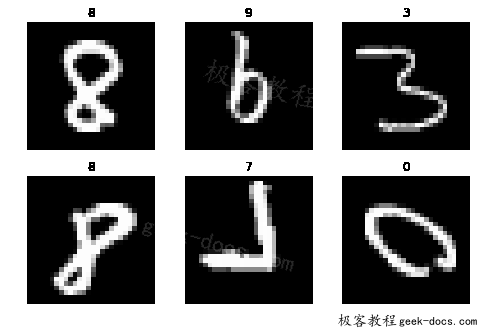## 影像特征标准化(Feature Standardization)

#对整个资料集进行"影像特征标准化" , mean=0, stdev=1
from  keras.datasets  import  mnist
from  keras.preprocessing.image  import  ImageDataGenerator
import  matplotlib.pyplot  as  plt

#在keras的backend预设使用tensorflow
#而tensorflow在处理图像是的资料结构是3维的张量(图像宽:width,图像高:height,图像频道:channel)

#载入数据
( X_train ,  y_train ),  ( X_test ,  y_test )  =  mnist . load_data ()

#将图像数据集的维度进行改变
#改变前: [样本数,图像宽,图像高] ->改变后: [样本数,图像宽,图像高,图像频道数]
X_train  =  X_train . reshape ( X_train . shape [ 0 ],  28 ,  28 ,  1 )
X_test   =  X_test . reshape ( X_test . shape [ 0 ],  28 ,  28 ,  1 )

#将像素值由"整数(0~255)"换成"浮点数(0.0~255.0)"
X_train  =  X_train . astype ( 'float32' )
X_test  =  X_test . astype ( 'float32' )

#定义"图像数据增强产生器(ImageDataGenerator)"的参数
datagen  =  ImageDataGenerator ( featurewise_center = True ,
featurewise_std_normalization = True )

#透过训练数据集来训练(fit)图像数据增强产生器(ImageDataGenerator)的实例
datagen . fit ( X_train )

#设定要"图像数据增强产生器(ImageDataGenerator)"产生的图像批次值(batch size)
# "图像数据增强产生器(ImageDataGenerator)"会根据设定回传指定批次量的新生成图像数据
for  X_batch ,  y_batch  in  datagen . flow ( X_train ,  y_train ,  batch_size = 9 ):
plt . figure ( figsize = ( 8 , 8 ))  #设定每个图像显示的大小
#产生一个3x3网格的组合图像
for  i  in  range ( 0 ,  6 ):
plt . subplot ( 331+ i )
plt . title ( y_batch [ i ])  #秀出图像的真实值
plt . axis ( 'off' )      #不显示坐标
plt . imshow ( X_batch [ i ] . reshape ( 28 , 28 ),  cmap = plt . get_cmap ( 'gray' ))

plt . show ()
break  #跳出回圈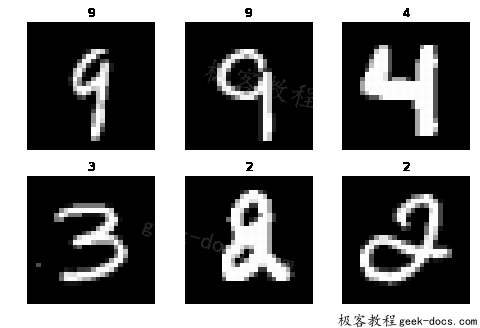## ZCA白化转换(ZCA Whitening)

# ZCA白化转换(ZCA Whitening)
from  keras.datasets  import  mnist
from  keras.preprocessing.image  import  ImageDataGenerator
import  matplotlib.pyplot  as  plt

#载入数据
( X_train ,  y_train ),  ( X_test ,  y_test )  =  mnist . load_data ()

#将图像数据集的维度进行改变
#改变前: [样本数,图像宽,图像高] ->改变后: [样本数,图像宽,图像高,图像频道数]
X_train  =  X_train . reshape ( X_train . shape [ 0 ],  28 ,  28 ,  1 )
X_test   =  X_test . reshape ( X_test . shape [ 0 ],  28 ,  28 ,  1 )

#将像素值由"整数(0~255)"换成"浮点数(0.0~255.0)"
X_train  =  X_train . astype ( 'float32' )
X_test  =  X_test . astype ( 'float32' )

#定义"图像数据增强产生器(ImageDataGenerator)"的参数
datagen  =  ImageDataGenerator ( zca_whitening = True )

#透过训练数据集来训练(fit)图像数据增强产生器(ImageDataGenerator)的实例
datagen . fit ( X_train )

#设定要"图像数据增强产生器(ImageDataGenerator)"产生的图像批次值(batch size)
# "图像数据增强产生器(ImageDataGenerator)"会根据设定回传指定批次量的新生成图像数据
for  X_batch ,  y_batch  in  datagen . flow ( X_train ,  y_train ,  batch_size = 9 ):
plt . figure ( figsize = ( 8 , 8 ))  #设定每个图像显示的大小
#产生一个3x3网格的组合图像
for  i  in  range ( 0 ,  6 ):
plt . subplot ( 331+ i )
plt . title ( y_batch [ i ])  #秀出图像的真实值
plt . axis ( 'off' )      #不显示坐标
plt . imshow ( X_batch [ i ] . reshape ( 28 , 28 ),  cmap = plt . get_cmap ( 'gray' ))

plt . show ()
break  #跳出回圈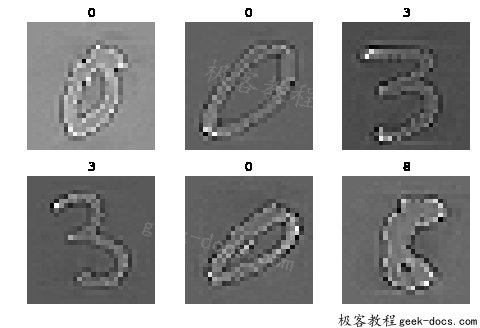## 储存增强的图像数据(Saving Augmented Images to File)

Keras允许您保存训练期间动态生成的图像。您可以在训练前指定给flow（）函数有关于您想保存的目录、文件名前缀和图像文件类型。然后在训练过程中，动态生成的图像将被写入到档案系统中。

#储存增强的图像数据(Saving Augmented Images to File)
from  keras.datasets  import  mnist
from  keras.preprocessing.image  import  ImageDataGenerator
import  matplotlib.pyplot  as  plt
import  os

#载入数据
( X_train ,  y_train ),  ( X_test ,  y_test )  =  mnist . load_data ()

#将图像数据集的维度进行改变
#改变前: [样本数,图像宽,图像高] ->改变后: [样本数,图像宽,图像高,图像频道数]
X_train  =  X_train . reshape ( X_train . shape [ 0 ],  28 ,  28 ,  1 )
X_test   =  X_test . reshape ( X_test . shape [ 0 ],  28 ,  28 ,  1 )

#将像素值由"整数(0~255)"换成"浮点数(0.0~255.0)"
X_train  =  X_train . astype ( 'float32' )
X_test  =  X_test . astype ( 'float32' )

#定义"图像数据增强产生器(ImageDataGenerator)"的参数
datagen  =  ImageDataGenerator ()

#透过训练数据集来训练(fit)图像数据增强产生器(ImageDataGenerator)的实例
datagen . fit ( X_train )

#产生要保存图像档案的目录
if  not  os . path . exists ( 'images' ):
os . makedirs ( 'images' )

#设定要"图像数据增强产生器(ImageDataGenerator)"产生的图像批次值(batch size)
# "图像数据增强产生器(ImageDataGenerator)"会根据设定回传指定批次量的新生成图像数据
for  X_batch ,  y_batch  in  datagen . flow ( X_train ,  y_train ,  batch_size = 9 ,  save_to_dir = 'images' ,  save_prefix = 'aug' ,  save_format = 'png' ):
plt . figure ( figsize = ( 8 , 8 ))  #设定每个图像显示的大小
#产生一个3x3网格的组合图像
for  i  in  range ( 0 ,  6 ):
plt . subplot ( 331 + i )
plt . title ( y_batch [ i ])  #秀出图像的真实值
plt . axis ( 'off' )      #不显示坐标
plt . imshow ( X_batch [ i ] . reshape ( 28 , 28 ),  cmap = plt . get_cmap ('gray' ))

plt . show ()
break  #跳出回圈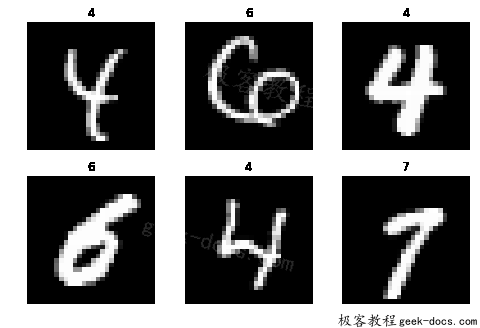## 用Keras增强图像数据的技巧

• 查看图像数据集花一些时间仔细检查你的数据集。看图像。注意可能有利于模型训练过程的图像准备和增强，例如需要处理场景中不同的轮班，旋转或翻转对象。
• 查看增强过的图像增强操作完成后，查看一些范例图像。理智地知道你正在使用什么样的图像变换是一回事，真正看到范例图像是非常不同的事情。查看您正在使用的单个增强图像以及您计划使用的全套增强图像。您可能会看到简化或进一步增强您的模型训练的方法。
• 评估一整套变换尝试多个图像数据准备和增强方案。通常情况下，您认为这样做不会有好处的转换,最后您可能会对数据增强后对模型的结果感到惊讶。

## 总结

• Keras中的ImageDataGenerator API可以用来动态产生新的转换后的图像来用于训练。
• 图像像素的标准化手法。
• ZCA白化转换。
• 对图像进行随机旋转，移动和翻转。
• 如何将转换后的图像保存到档案系统中以备后用。

• 回顶